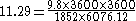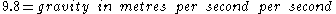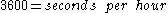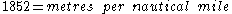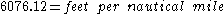xROTEncyclopedia
The aviation
Aviation
Aviation is the design, development, production, operation, and use of aircraft, especially heavier-than-air aircraft. Aviation is derived from avis, the Latin word for bird.-History:...

term ROT stands for rate one turn, also known as a standard rate turn. All aircraft
Aircraft
An aircraft is a vehicle that is able to fly by gaining support from the air, or, in general, the atmosphere of a planet. An aircraft counters the force of gravity by using either static lift or by using the dynamic lift of an airfoil, or in a few cases the downward thrust from jet engines.Although...

must be able to perform a standard rate turn.

A standard rate turn for (light) airplanes is defined as a 3° per second turn, which completes a 360° turn in 2 minutes. This is known as a 2-minute turn, or rate one (= 180°/minute).

For heavy airplanes a standard rate turn is a 4-minute turn.

Instruments, either the turn and bank indicator
Turn and bank indicator
In aviation, the turn and bank indicator shows the rate of turn and the coordination of the turn. The rate of turn is indicated from a rate gyroscopically and the coordination of the turn is shown by either a pendulum or a heavy ball mounted in a curved sealed glass tube. No pitch information is...

or the turn coordinator
Turn coordinator
The turn coordinator is a flight instrument which displays to a pilot information about the rate of yaw , roll, and the coordination of the turn...

, have the standard rate turn clearly marked. Light aircraft are equipped with 2-minute turn indicators while heavy aircraft are equipped with 4-minute turn indicators. This is very useful to pilots who are out of visual contact with the ground and for air traffic control when appropriate separation of aircraft is desired. The pilot banks the airplane such that the turn and slip indicator points to the standard rate turn mark and then uses a watch to time the turn. The pilot can pull out at any desired direction depending on the length of time in the turn.

A rate half turn (1.5° per second) is normally used when flying faster than 250 kt. The term rate two turn (6° per second) used on some low speed aircraft.

Angle of Bank formula

The formula for calculating the angle of bank for a specific True Airspeed
True airspeed
True airspeed of an aircraft is the speed of the aircraft relative to the airmass in which it is flying. True airspeed is important information for accurate navigation of an aircraft.-Performance:...

(TAS) is: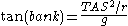whereis the radius of the turn and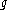is the acceleration due to gravity.
For a rate one turn and velocity in kt (nautical miles per hour), this comes to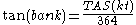.

A convenient approximation for the bank angle in degrees is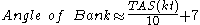One might also want to calculate the radius of a Rate 1,2 or 3 turn at a specific TAS.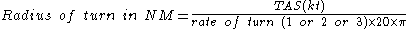or in SI units,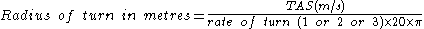Use this formula to calculate the radius of turn in feet given velocity in knots and angle of bank: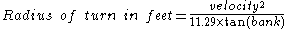This is a simplified formula that ignores slip and returns zero for 90 degrees of bank.

The constant 11.29 is calculated: